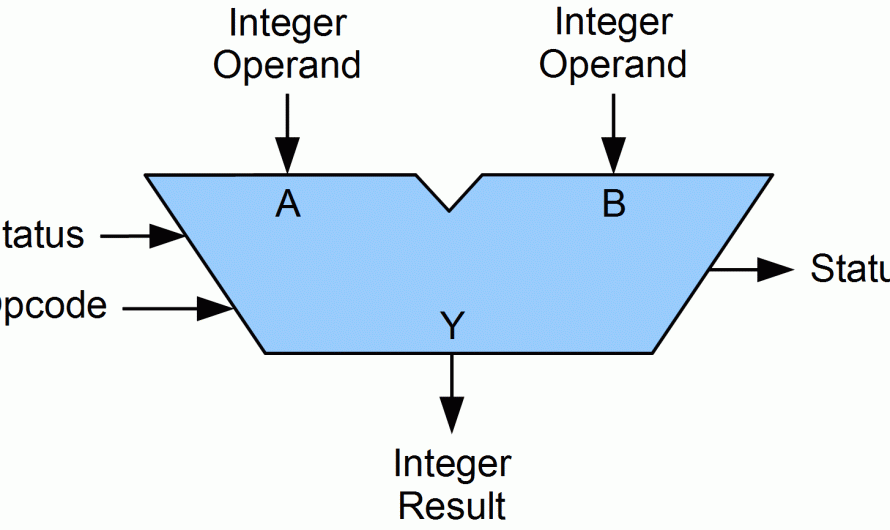December 7, 2023# What Is An Arithmetic Logic Unit (ALU) and Its Functions?

Hello there, in this article we will discuss the Arithmetic Logic Unit (ALU) and its functions. ALU is one of the most important components of computing circuits like CPUs and GPUs.

ALU usually handles the mathematical operations that processing units receive. Dive down in this article to know more about the ALU and why are they so important.

And don’t forget to watch the bonus video at the end of the article.

## What is the APU?

An arithmetic logic unit (ALU) is a digital electronic circuit present within the CPU that performs arithmetic and bitwise operations.

ALU deals with integer binary numbers while the floating point unit (FPU) deals with floating point numbers. ALU is a fundamental building block of many types of computing circuits like CPUs and GPUs.

A single CPU or GPU may also consist of more than one ALU. Moreover, input to the APU is the data to be operated called operand.

When the operation becomes more complex, then the ALU also becomes more expensive and takes up more space in the CPU and also produces more heat.

That’s why ALUs are powerful enough to ensure that the CPU fast but not so complex to become very expensive.

##Related: What Is A CPU? | CPU Functions, Components, And Diagram

## Arithmetic Logic Unit (ALU) Signals

An Arithmetic Logic Unit (ALU) has a variety of input and output electrical connections that helps to convey digital signals between the ALU and external electronics.

External circuits give signals to the ALU input and in response, ALU outputs signal to external electronics.

#### Data:

ALU has three parallel buses consisting of two input operands and an output operand. The number of signals handled by all the three buses is the same.

Every data bus is a group of signals that conveys one binary integer number.

#### Opcode:

The opcode input is a parallel bus that transmits an operation selection code to the ALU. An operation selection code is a calculated value that specifies the desired arithmetic or logic operation that an ALU is going to perform.

The opcode size determines the greatest number of various operations the ALU can perform.

#### Status:

Output: The status outputs are many individual signals that convey supplemental data about the results of the ALU operations.

General ALUs usually have status signals such as: Carry out, zero, negative, overflow, etc. After the completion of each ALU operation, the status output signals were in external registers.

Storing these signals in registers makes them available for future ALU operations.

Input: The status inputs permit further information to access to the ALU once performing an operation. Moreover, this is a single “carry-in” bit that’s the stored carry-out from a previous ALU operation.

##Related: What’s the difference between CPU and GPU?

## Arithmetic Logic Unit (ALU) Functions

Arithmetic Logic Units (ALU) has various numbers of basic arithmetic and bitwise logic functions. General purpose ALUs usually include these operations:

Arithmetic Operations: Arithmetic operations include addition and subtraction. However, sometimes multiplication and division are also used, these operations are more expensive to make.

That’s why multiplication and division are done by repetitive addition and subtraction. Some common arithmetic operations that an ALU performs are: add, subtract, increment, decrement, etc.

Bit shift operations: Bit shift operations cause operand to shift left or right. Simple ALUs can shift operands by only one-bit position, whereas more complex ALUs can shift by many bits.

Complex ALUs are able to shift by many bits because they have barrel shifters.

Bitwise Logical Operations: These are the operations like AND, OR, NOT, NOR, NAND, etc.